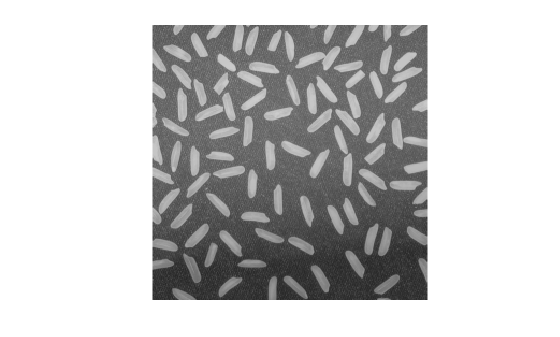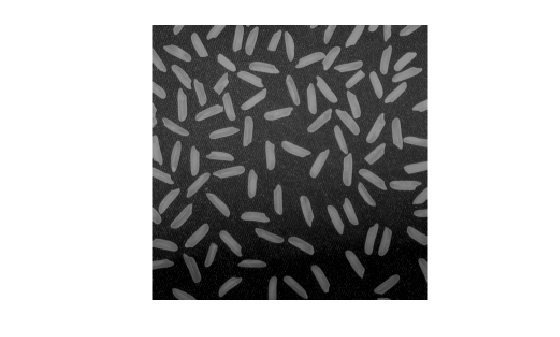# imsubtract

Subtract one image from another or subtract constant from image

## Syntax

``Z = imsubtract(X,Y)``

## Description

example

````Z = imsubtract(X,Y)` subtracts each element in array `Y` from the corresponding element in array `X` and returns the difference in the corresponding element of the output array `Z`.```

## Examples

collapse all

This example shows how to subtract two `uint8` arrays. Note that negative results are rounded to 0.

```X = uint8([ 255 0 75; 44 225 100]); Y = uint8([ 50 50 50; 50 50 50 ]); Z = imsubtract(X,Y)```
```Z = 2x3 uint8 matrix 205 0 25 0 175 50 ```

Read a grayscale image into the workspace.

`I = imread('rice.png');`

Estimate the background.

`background = imopen(I,strel('disk',15));`

Subtract the background from the image.

`J = imsubtract(I,background);`

Display the original image and the processed image.

`imshow(I)````figure imshow(J)```Read an image into the workspace.

`I = imread('rice.png');`

Subtract a constant value from the image.

`J = imsubtract(I,50);`

Display the original image and the result.

`imshow(I)````figure imshow(J)```## Input Arguments

collapse all

First array (minuend), specified as a numeric array or logical array of any dimension.

Second array (subtrahend) to be subtracted from `X`, specified as a numeric array or logical array of the same size and class as `X`, or a numeric scalar of type `double`.

## Output Arguments

collapse all

Difference, returned as a numeric array of the same size as `X`. `Z` is the same class as `X` unless `X` is logical, in which case `Z` is data type `double`. If `X` is an integer array, then elements of the output that exceed the range of the integer type are truncated, and fractional values are rounded.